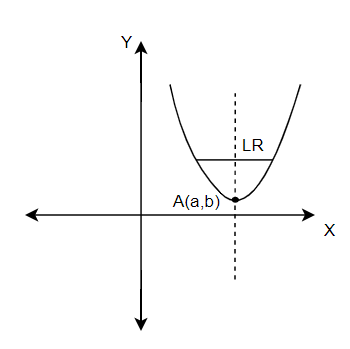QuestionAnswers

# The vertex of a parabola is the point $\left( a,b \right)$ and the latus rectum is of length $l$. If the axis of the parabola is along the positive direction of $y-$axis, then its equation is(a) ${{\left( x-a \right)}^{2}}=\dfrac{l}{2}\left( y-2b \right)$(b) ${{\left( x-a \right)}^{2}}=\dfrac{l}{2}\left( y-b \right)$(c) ${{\left( x-a \right)}^{2}}=l\left( y-b \right)$(d) None of theseVerified
155.4k+ views
Hint: The form of the parabola to be used in the questions is ${{\left( x-{{x}_{1}} \right)}^{2}}=4a\left( y-{{y}_{1}} \right)$.

The vertex and the latus rectum of a parabola are given as $\left( a,b \right)$ and $l$ respectively in the question.
Since the axis of the parabola is along the positive direction of the $y-$axis, we can figure out that the form of the required parabola would be ${{x}^{2}}=4ay$. Latus rectum is indicated by LR and the given point of the vertex is termed as A. We can represent the details as shown in the figure below.The vertex, A is $\left( a,b \right)$, so we can write the equation for the parabola as,
${{\left( x-a \right)}^{2}}=4c\left( y-b \right)\ldots \ldots \ldots (i)$
Since the coordinate of the vertex is $a$, the term $c$ has been used in the equation above.
From the figure, we can see that the latus rectum is perpendicular to the axis of the parabola and is represented in the figure as LR. We know that the length of the latus rectum for the form of parabola, ${{x}^{2}}=4ay$ is $4c$. Also, it is already given to us in the question as $l$.
Therefore, we can relate the data and we can write the term $4c=l$.
After substituting this relation in equation $(i)$, we get the equation of the parabola as,
${{\left( x-a \right)}^{2}}=l\left( y-b \right)$
Hence, option (c) is obtained as the correct answer.

Note: The best way to approach this question is to figure out the form of the required equation. Looking at the options, the form of the parabola can be obtained easily. One way to figure out the answer would be to check the latus rectum. Since the length of the latus rectum is available from the question, the answer can be computed easily in less time.